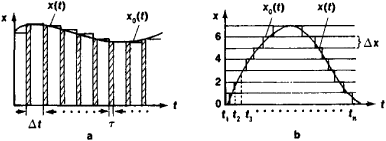# Quantization, Signal

## Quantization, Signal

discretization of analogue signals, the conversion of an electric signal, continuous in time and level, into a succession of discrete or discrete-analogue signals that in aggregate represent the original signal with a predetermined error. Signal quantization is used in telemechanics for data transmission, in computer engineering for analogue-to-digital conversion, and in automatic pulse systems.

In the transmission of analogue signals it is usually sufficient to transmit not the signal itself but a succession of its instantaneous values, taken from the original signal according to a particular principle. Signals can be quantized with reference to time or to level or simultaneously to both parameters. If done with reference to time, the signal is either interrupted (pulse signal) or changed abruptly (stepwise signal; see Figure 1) at equal time ΔtFigure 1. Signal quantization (a) by time and (b) by level; x0(t) is the original signal; X(t) is the quantized signal; Δt is the quantization interval; ΔX is the quantization level

For example, an analogue signal passing through the contacts of a periodically switched electric relay is converted to a succession of pulse signals. For infinitely small switching intervals (on or off), that is, for an infinitely large contact-switching frequency, an accurate representation of the original signal is obtained. If signals are quantized with reference to level, the instantaneous values of the analogue signal are replaced respectively by the nearest discrete levels, which form a discrete scale of quantization. Any value of the signal falling between levels is rounded off to the value of the nearest level. For an infinitely large number of levels, a quantized signal is converted to the initial analogue signal.

### REFERENCES

Kharkevich, A. A. Bor’ba s pomekhami, 2nd ed. Moscow, 1965.
Marcuse, J. Diskretizatsiia i kvantovanie. Moscow, 1969. (Translated from French.)

M. M. GEL’MAN

Mentioned in ?
References in periodicals archive ?
In , the quantized state estimation problem for discrete-time linear systems has been addressed; the work in  considered the [H.sub.[infinity]] filtering problem for a class continuous-time polytopic uncertain systems subject to measurement quantization, signal transmission delay, and data packet dropout; the problem of [H.sub.[infinity]] filtering for discrete-time T-S fuzzy systems with measurement quantization and packet dropouts has been studied in ; and  investigated the problem of [H.sub.[infinity]] filtering for discrete-time polytopic uncertain systems with measurement quantization; in , the quantized [H.sub.[infinity]] filtering problem for a class of discrete-time polytopic uncertain systems with packet dropout has been considered.
Topics cover the characterization of continuous images, sampling and quantization, signal processing, enhancement and restoration, analysis, and software implementation.

Site: Follow: Share:
Open / Close